# Investigation on the Correlation of CFD and EFD Results for A Supercritical Wing

Investigation on the Correlation of CFD and EFD Results for A Supercritical Wing

Dawei LiuDehua Chen Qiang Li Xin Xu Xin Peng

State Key Laboratory of Aerodynamics, Mianyang, China, 621000

High Speed Aerodynamics Institute, China Aerodynamics Research and Development Center, Mianyang, China, 621000

Corresponding Author Email:
liudawei@mail.ustc.edu.cn
Page:
19-26
|
DOI:
http://doi.org/10.18280/ijht.330303
N/A
|
Accepted:
N/A
|
Published:
30 September 2015
| Citation

OPEN ACCESS

Abstract:

The correlation of EFD (experimental fluid dynamics) and CFD (computational fluid dynamics) results for a typical supercritical wing was investigated to predict its aerodynamic characteristics well in this paper. A wind tunnel test was conducted in European Transonic Wind tunnel (ETW) to obtain the pressure distribution on a typical supercritical wing test model; also integrated lift force coefficients and pitching moment coefficients were achieved. Computational results were obtained through the debugged RANS solver based on the experimental results. It was showed that a better correlation of EFD and CFD pressure distribution could be achieved, through debugging the CFD method with EFD results, including investigations of grid divergence, turbulence model influence and test model deformation simulation. Test model deformation simulation could apparently improve the correlation of pressure distribution for outboard wing sections. The optimization of turbulence model parameters based on the generic algorithm could improve the correlation of CL~α curve at small angles, but the prediction of separation point was poorly correlated with EFD results. However, the turbulence model parameters optimization implied a promising way to further improve the correlation for supercritical wing especially for multipoint optimization.

Keywords:

Correlation, CFD, EFD, Supercritical wing, Grid convergence, Turbulence model parameters, Optimization.

1. Introduction

It was a main method to obtain the aerodynamic characteristics of aircraft and aerospace vehicles, through the experimental techniques using wind tunnels or water tunnels (experimental fluid dynamics: EFD) until 1970’s. In the past forty years, the computational fluid dynamics has played a more and more important role in the aerodynamic prediction with the progress of the computer hardware and computational algorithm. At present, the CFD are widely used in the aircraft configuration optimization in the primary design stage for its high efficiency and low cost with the comparison of EFD. However, EFD and CFD are usually conducted separately by different groups of experts with weak interaction and collaboration, thus the correlation study of EFD and CFD may be expected to further improve the CFD prediction .

For the high cruise efficiency, the supercritical wing was widely used in the civil transport aircraft as airbus A350 and Boeing aircraft 777. The supercritical wing has been developed for more than forty years, and the supercritical wing has a plump leading edge and rather flat upper surface [2-4]. The velocity of the flow reduced to zero at stagnation point near leading edge, then rapidly increased to sound speed on the upper wing surface at cruise condition. In most cases, then flow velocity will further increase to supersonic until the shock wave occurs on the wing. The shock wave terminated the supersonic areas, and sometimes induced the boundary layer separation. The whole flow around the upper wing surface is close to sonic flow, which is very sensitive and complex, including cross flow and shock induced boundary layer separation. The results of different turbulence model and grids adopted varied much [2,3]. So, it is a great challenge to predict the aerodynamic characteristic of a supercritical wing using CFD method. Mostly, the EFD method could predict the aerodynamic performance well, however, factors like the existence of support system, the difference of the magnitude Reynolds number, the vibration and deformation of test model would all cause some interference to the EFD data [4-6]. Thus, the correlation of CFD and EFD turns difficult.

In this paper, a wind tunnel test was conducted in European Transonic Wind tunnel (ETW) to obtain the pressure distribution on a typical supercritical wing test model; also integrated lift force and pitching moment coefficients were achieved. Test results was used to debug the CFD method for the correlation improvement of EFD and CFD results, including investigations of the grid divergence, the influence of different turbulence model, the optimization of turbulence model parameters based on the generic algorithm and the simulation of test model deformation.

2. Wind Tunnel Test

2.1 Test model

The test model is combined with a supercritical wing and a typical transport aircraft fuselage, the wing span is 1.56m. The model was made from maraging steel for cryogenic conditions. There are 9 wing span sections where pressure orifices were located, and the exact location was shown in the figure 1. The test model adopted artificial fixed transition at low Reynolds Number. The transition trip was 25mm away from the nose on the fuselage (see figure 2), and the 7% local chord length away from the leading edge on the wing. The test model was installed in test section by the Z sting support.

## 1.pngFigure 1. The simplified sketch of pressure orifices location (y/b)

## 2.png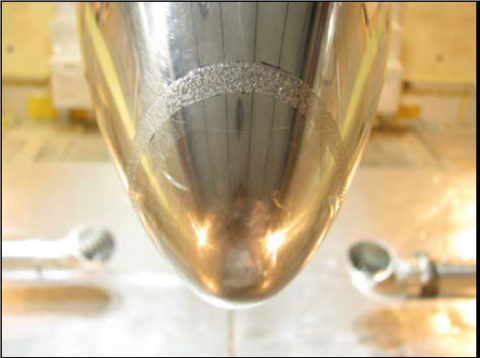Figure 2. The transiton trip on the fuseluge nose of test model

2.2 Facilities

The ETW is a unique facility that enables testing of aircraft configurations at conditions ranging from subsonic to low supersonic speeds at Reynolds numbers up to full-scale flight values (seen in figure 3 and figure 4). It is a cryogenic, closed circuit, continuous-flow, fan-driven wind tunnel. The capability of varying the gas temperature, pressure and speed independently allows for pure Reynolds number and/or aeroelastic investigations [7-9].

The test section dimensions are 2.4m in width, 2m in height and about 9m in length. The ETW operating range covers pressures from 110kPa to 450kPa and temperatures from 313K down to 110K allowing the achievement of maximum Reynolds numbers of 50 million for full models and 90 million for semispan models at a Mach number around 0.85.

## 3.png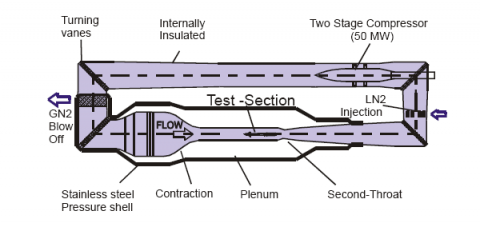Figure 3. The composition sketch of ETW wind tunnel

## 4.png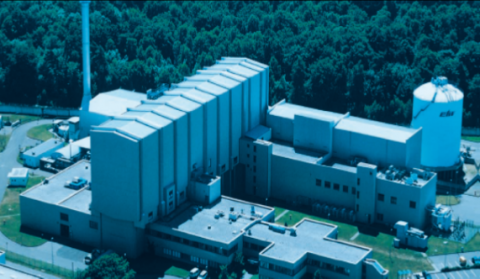Figure 4. The photo of ETW wind tunnel

2.3 Test conditions and data correction

For this model, the Mach numbers ranged from 0.40 to 0.86, nominal incidence angles from -4° to 12°, and the Reynolds numbers from 2.3×106 to 3.5x107 based on the mean aerodynamic chord length. To achieve the varieties of Reynolds numbers, the total pressure ranged from 124 kPa to 340 kPa, and the total temperature from 114K to 300K. Most of the data were offered in continuous form.

All corrections were applied in accordance with the guidelines provided in the Technical Memorandum “Tunnel Corrections in ETW”, mainly including Mach number correction, wall interference correction and thermal contraction correction due to the cryogenic conditions.

3. CFD Method

3.1 Governing equation

3D non-dimensional Navier-Stokes equations were as the following,

$\frac{\partial Q}{\partial t}+\frac{\partial F}{\partial \xi}+\frac{\partial G}{\partial \eta}+\frac{\partial H}{\partial \zeta}=\frac{1}{\operatorname{Re}}\left(\frac{\partial F_{v}}{\partial \xi}+\frac{\partial G_{v}}{\partial \eta}+\frac{\partial H_{v}}{\partial \zeta}\right)$     (1)

Detailed description on the equations could be found in reference [9, 10].

3.2 Boundary conditions

The velocity, pressure and temperature boundary conditions were as the following.

$\left\{\begin{array}{c}\mathrm{r} \\ V=0 \\ \frac{\partial P}{\partial n}=0 \\ \frac{\partial T}{\partial n}=0\end{array}\right.$   (2)

While the inlet and outlet boundary conditions were:

$\left\{\begin{array}{c}\rho=\left(\frac{\rho_{\infty}^{\gamma} a^{2}}{\gamma P_{\infty}}\right)^{\frac{1}{\gamma-1}} \\ \vec{V}=\vec{V}_{\infty}+\left(V_{n}-V_{\infty}\right) \vec{n} \\ P=P_{\infty}\left(\frac{\rho}{\rho_{\infty}}\right)^{\gamma}\end{array}\right.$   (3)

$\left\{\begin{array}{c}\rho=\left(\frac{\rho_{e}^{\gamma} a^{2}}{\gamma P_{e}}\right)^{\frac{1}{\gamma-1}} \\ \vec{V}=\vec{V}_{e}+\left(V_{n}-V_{e}\right) \vec{n} \\ P=P_{e}\left(\frac{\rho}{\rho_{e}}\right)^{\gamma}\end{array}\right.$    (4)

Symmetry boundary conditions were adopted in the symmetry surfaces of grid.

3.3 Governing equations solution method

A three dimensional Reynolds averaged Navier-Stokes flow solver that works on structured multiblock grids is developed. The equations are cast in a cell-centered finite-volume form and the convective flux calculation follows Osher’s approximate Riemann solver with a MUSCL scheme for higher order accuracy. An implicit method is used for the time discretization. This solver has high precision and good stability, and can control the oscillation of the results effectively.

The local time step was used in this article to accelerate convergence, and the turbulence model influence was investigated, including Abid k-ε (k-epsil), Menter k-ω SST (sst), Spalart-Allmaras (S-A) and Wilcox k-ω (K-omg) turbulence model. The description of the turbulence model could be seen in the reference .

3.4 CFD solver validation

The flow over Ty154 stand model was simulated using the CFD solver developed in this paper. This stand model was used to check the system of FL-26 wind tunnel before test in China Aerodynamic Research and Development Center (CARDC), which was made in metal and came from the simplified shape of the civil plan Ty154 of Russia. The model comprised a fuselage, clean wing and a T empennage.

The longitudinal aerodynamic characteristics of this model were computed to validate the CFD solver. Figure 5 shows the computational grids over the model surface and the girds topology are shown in the Figure 6. Typical comparison results at M=0.8 were shown in Figure 7. It can be seen that the slopes of CL~α and Cm~α curves are nearly the same for EFD and CFD results, also the break points are at the same incidence angles. The shape of two CD~ α curves are similar, and the CFD drag coefficients are a little bigger than EFD results, the possible reasons maybe the uncorrected sting support interferences, which also contributed to the Cm differences of Cm~α curve. However, the EFD results compared well with CFD results in whole, implying the CFD solver reliable.

## 5.pngFigure 5. Grids distribution on the model surface

## 6.png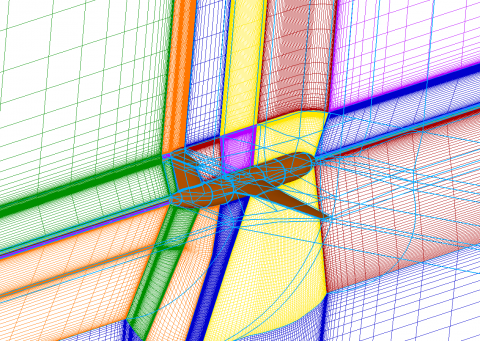Figure 6. Grids topology of Ty154 model

## 7a.png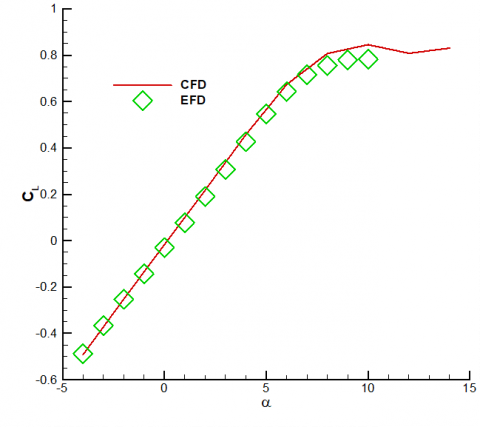(a) CL~α

## 7b.png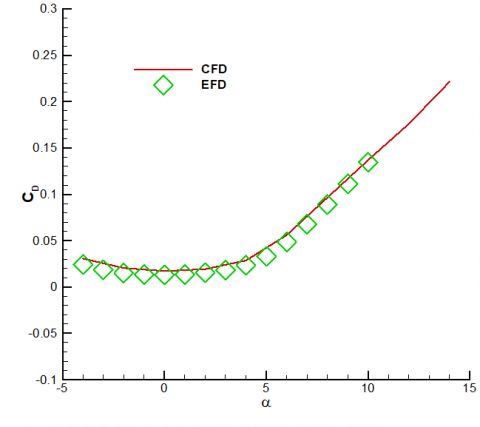(b) CD~α

## 7c.png(c) Cm

Figure 7. Comparision between EFD and CFD results of Ty154 model (M=0.8)

4. Correlation Improvement of CFD and EFD

4.1 Grid convergence

Numerical results are dependent of the computational grids, especially for the coarse grids. Different results will be obtained while using different magnitude grids. In theory, when the grids magnitude is large enough, computational results will converge, and the results are independent of the grids magnitude. However, the grids magnitude cannot be very large with the limit of computer resources, thus if the difference due to grids magnitude effects are thought to be acceptable in engineering, the girds will be suitable.

Four set of grids were generated to study the grid convergence in this paper. The grid numbers are 2 million, 4 million, 10 million and 20 million, and the grids distribution could be seen in the table 1. Four set of grids were kept the same topology as seen in the figure 8.

Table 1. Different grids magnitude for CFD model

 stream  wise span wise normal trailing  edge girds amount small 141 73 69 9 2 M medium 191 99 73 13 4 M large 297 129 105 17 10 M largest 359 175 113 21 20 M

## 8.png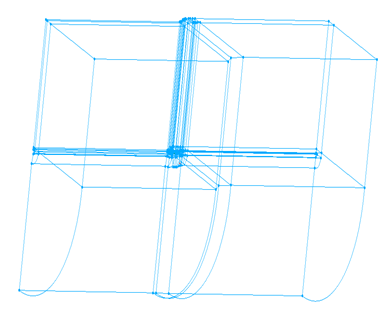Figure 8. Grids Topology of the supercritical wing

## 9.png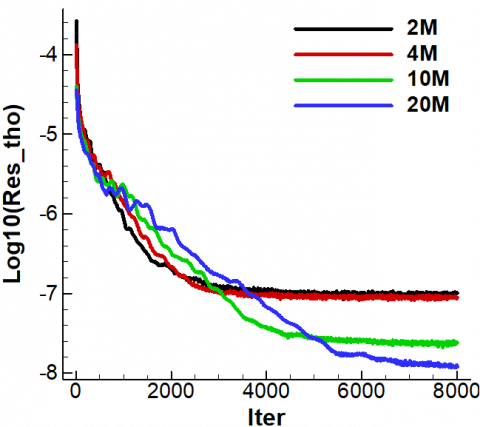Figure 9. Residual lines of different gird numbers

Flow over the supercritical wing was solved for different computational grids using the CFD solver developed in this paper at M=0.76, α=2° and Rec=3.3 million. Figure 9 showed the residual lines of different grid numbers, and the grids of 2 million and 4 million converged fast while the grids of 10 million and 20 million converged much more slowly. Figure 10 displayed the grids convergence of longitudinal aerodynamic characteristics of the supercritical wing. It was obvious that the aerodynamic characteristics changed abruptly with the grids as grids number below the 10 million. The aerodynamic characteristics varied much more gently when the grids number increasing from 10 million to 20 million. Table 2 presented the converged grids solution for the supercritical wing. The extrapolated solution equals the computational results while the grids number is infinitely large. It can be seen that the largest gird’s solution was the most close to the extrapolated results, and the large grid’s solution did not appear large difference with the extrapolated results. However, the largest grids cost much more computer resources than the large grids. To compensate the solution reliability and computational efficiency, the large grids was selected to solve the flow over the wing in this paper.

## 10a.png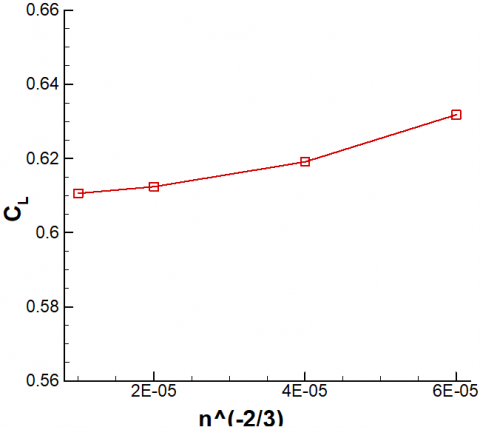## 10b.png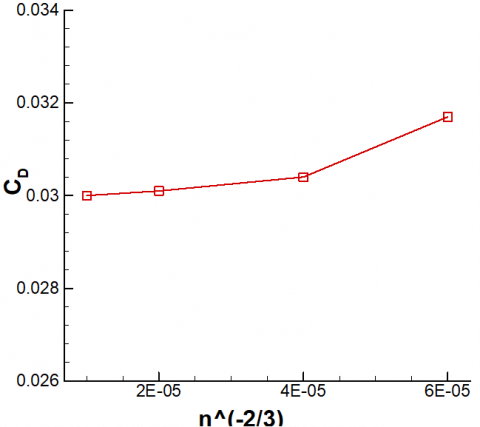## 10c.pngFigure 10. Grids convergence of longitudinal aerodynamic characteristics (M=0.76, α=2°)

Table 2. Converged grids solution for a supercritical wing (M=0.76, α=2°)

 CL ∆CL CD ∆CD Cm ∆Cm n-2/3(10-5) small 0.6318 0.0241 0.0317 0.0019 -0.1573 -0.0084 6.1 mideum 0.6191 0.0114 0.0304 0.0006 -0.1533 -0.0044 3.9 large 0.6164 0.0047 0.0304 0.0003 -0.1530 -0.0029 2.1 largest 0.6106 0.0029 0.0300 0.0002 -0.1507 -0.0018 1.3 extrapolated 0.6077 / 0.0298 / -0.1489 / 0.0

4.2 Turbulence model influence

Numerical simulated results will be influenced by different turbulence models while using the RANS solver. The aerodynamic characteristics of the wing were numerically investigated with different turbulence models, including Abid k-ε (k-epsil), Menter k-ω SST (sst), Spalart-Allmaras (S-A) and Wilcox k-ω (K-omg) at M=0.76, α=2° and Rec=6.6 million.

It was shown in the figure 11 that the pressure distribution comparison between the EFD results and the CFD results while utilizing different turbulence models for different span wise location. The pressure distribution compared well with the CFD results except shock wave location for the inboard location wing sections (η=11.32%, η=22.23%), while the agreement of EFD and CFD results was not so good for outboard sections (η=54%, η=63%). The CFD pressure coefficients were a little minus than EFD results before the shock wave location for outboard sections, for the reason that test model deformation was not simulated. The minus twist angles of outboard sections resulted in a lower local incidence angle. For the inboard sections, test model deformation was much smaller due to its higher thickness.

As a whole, the influences of different turbulence models were not so apparent except the shock wave location, and the sst turbulence model showed much more advantage in capturing the shock location than other turbulence models. Therefore, sst turbulence model was used to close the N-S equation.

## 11a.png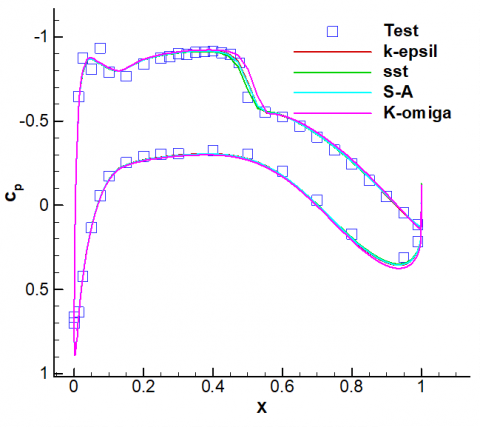(a) η=11.32%

## 11b.png(b) η=22.23%

## 11c.png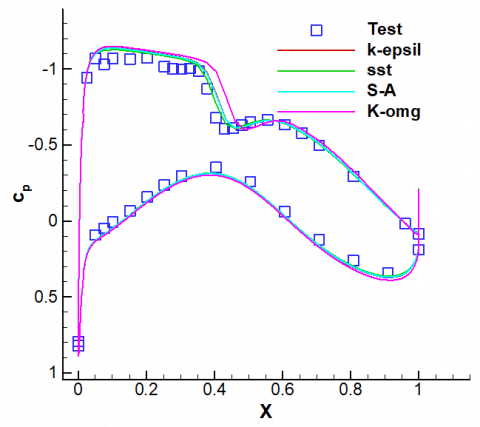(c) η=54%

## 11d.png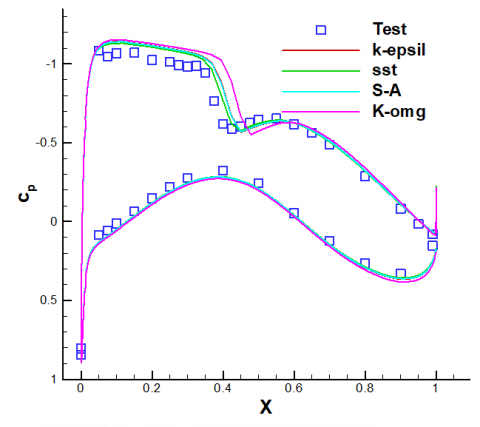(d) η=63%

Figure 11. Pressure distribution comparison between the EFD results and the CFD results utilizing different turbulence models (M=0.76, α=2°, Rec=6.6×106)

4.3 Test model deformation simulation

As discussed previously in this paper, the model deformation during the wind tunnel test caused disagreement of EFD and CFD results for outboard wing sections. To seek a much better correlation of EFD and CFD results, the test model deformation was measured. The deformed model was simulated based on the grids deformation technology.

Figure 12 showed the pressure distribution comparison between the EFD results and the CFD results for deformed and undeformed models at M=0.76, α=2° for different span wise location. It was obviously seen that the correlation of EFD and CFD pressure distribution greatly improved for outboard wing sections. Test model deformation must be considered for a better correlation of EFD and CFD results for the supercritical wing.

## 12a.png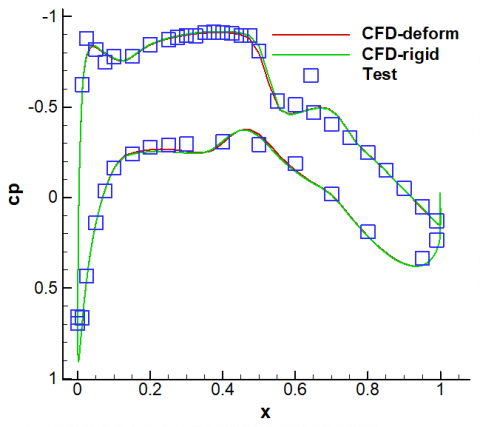(a) η=11.32%

## 12b.png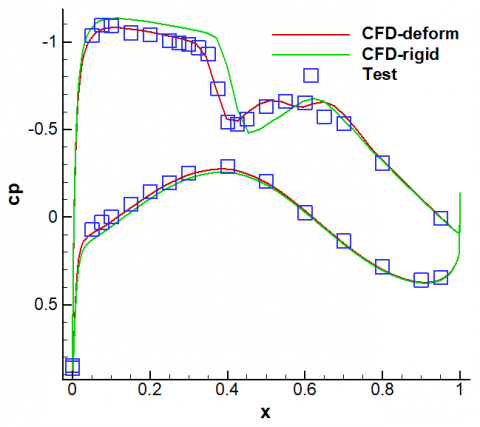(b) η=54%

## 12c.png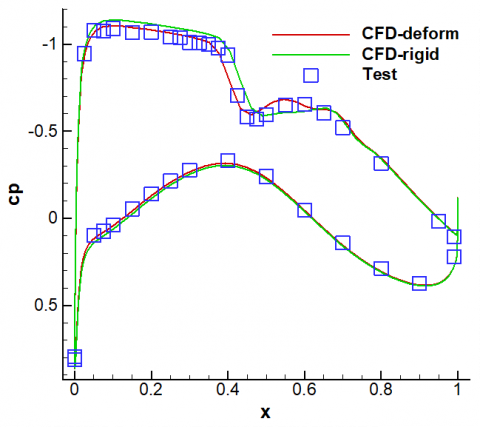(c) η=72.36%

## 12d.png(d) η=86.1%

Figure 12. Pressure distribution comparison between the EFD results and the CFD results for deformed and undeformed models (M=0.76, α=2°)

4.4 Turbulence parameters optimization based on the genetic algorithm

The pressure distribution correlation of EFD and CFD achieved a good agreement for the supercritical wing after several investigations. However, the CL~α curve integrated with EFD pressure distribution did not correlate well with CFD results as seen in the Figure 13. The slopes of two curves were nearly the same, but the lift coefficient of CFD was higher than EFD results at a constant incidence angle, possibly for the accumulation differences of wing section’s pressure distribution. Therefore, the study on turbulence parameters optimization was tried to improve the lift coefficient correlation. The selected k-ω sst turbulence model developed by Menter, was a mixed two equation model, for which the k-ω model was applied near the wall and the k-ε model was utilized far away the wall. It can simulate the flow with strong adverse pressure gradient well and be widely used in the engineering. For this model, there are two groups of parameters obtained through experiment and theoretical computation at special conditions. In most cases, the original parameters of the turbulence could achieve good results, but varieties of turbulence parameters may reach further improved simulation. Generic optimization method was used to seek the optimal turbulence parameters efficiently, and the optimal target was the lift coefficient difference of EFD and CFD. The single point optimization problem could be described as,

Design point: M=0.76, $\alpha$ =2 °, Re=3.3E+06

Design target: min {│CL_CFD-CL_EXP│}

Design parameters: nine turbulence parameters, including β*, κ, σk1, σω1, β1, a1, σk2, σω2 and β2. The range of these parameters could vary 30% in original value, seen in the table 3.

## 13.png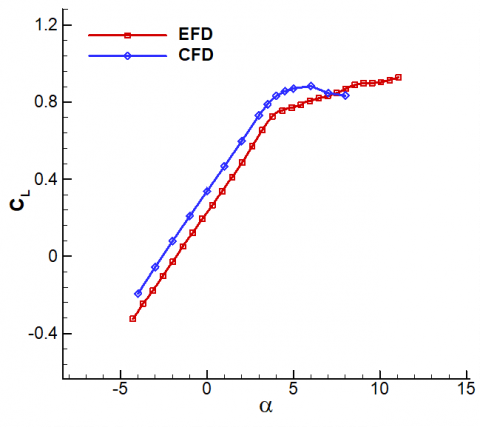Figure 13. EFD and CFD results comparison for CL~α curve

Table 3. Range of the optimization parameters

 β* κ σk1 σω1 β1 a1 σk2 σω2 β2 xmin 0.072 0.328 0.68 0.4 0.06 0.248 0.8 0.6848 0.0662 xorig 0.09 0.41 0.85 0.5 0.075 0.31 1.0 0.856 0.0828 xmax 0.108 0.492 1.02 0.6 0.09 0.372 1.2 1.0272 0.0994

Figure 14 showed the variety of target CL with the iteration steps. It could be seen that the optimization result converged at the iteration 6, and lift coefficient differences of EFD and CFD at design point became small. Then, the optimized CFD results CL~α curve were obtained with the optimal parameters. Figure 15 displayed the comparison of EFD results, original CFD results and optimized CFD results. It is not hard to see that optimized CFD results compared better with EFD results than original CFD results at lower angle, but the separation occurred too early which seemed to be unreasonable. Though optimized results may be unreasonable, it directed a promising way to further improve the correlation of CFD and EFD for a supercritical wing, especially with multiple target points optimization.

## 14.png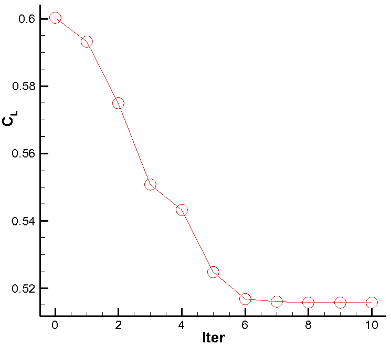Figure 14. The optimization of CL converged history

## 15.pngFigure 15. The EFD and optimized CFD results comparison for CL~α curve

5. Conclusion

To study the aerodynamic characteristics of a supercritical wing, wind tunnel test and CFD simulation were both conducted in this paper. A better correlation of EFD and CFD pressure distribution could be achieved, through debugging the CFD method with EFD results, including investigations of grid divergence, turbulence model influence and test model deformation simulation. Test model deformation simulation could apparently improve the correlation of pressure distribution for outboard wing sections. The optimization of turbulence model parameters based on the generic algorithm was used to improve the CL~α curve correlation. The optimized CFD results showed a much better correlation with EFD results at small angles, but the prediction of separation point was poorly correlated with EFD results. However, the turbulence model parameters optimization showed a promising way to further improve the correlation for supercritical wing especially for multipoint optimization.

Nomenclature
 α Angle of attack T CL Temperature Lift coefficient CD Drag coefficient Cm Pitching moment coefficient P Pressure ρ Density T Temperature V Velocity
References

1.    Hideji Saiki and Jyun Ogino, Towards EFD/CFD Integration: Development of DAHWIN-Digital/Analog-Hybrid Wind Tunnel, AIAA 2014-0982. DOI: 10.2514/6.2014-0982.

2.    Xiaoquan Gong, The Effects of Turbulence Model Corrections on Drag Prediction of NASA Common Research Model, AIAA 2014-3171. DOI: 10.2514/6.2014-3171.

3.    Leonardo Scalabrin, Grid Assessment Using the NASA Common Research Model (CRM) Wind Tunnel Data, AIAA 2013-0052. DOI: 10.2514/6.2013-52.

4.    Makoto Ueno, 80% Scaled NASA Common Research Model Wind Tunnel Test of JAXA at Relatively Low Reynolds Number, AIAA 2013-0493, 2013. DOI: 10.2514/6.2013-493.

5.    Melissa B. Rivers, Support System Effects on the NASA Common Research Model, AIAA 2012-0707. DOI: 10.2514/6.2012-707.

6.    Masataka Kohzai, Wall and Support Interference Corrections of NASA Common Research Model Wind Tunnel Tests in JAXA, AIAA 2013-0963. DOI: 10.2514/6.2013-963.

7.    LIU Dawei and WANG Yuanjing, Numerical Investigation on the Reynolds Number Effects of Supercritical Airfoil, ACMS 2011, v 31, p103-109, 2012. DOI: 10.1016/j.proeng.2012.01.998.

8.    Karl Pettersson, Arthur Rizzi. Aerodynamic Scaling to Free Flight Conditions: Past and present, Progress in Aerospace Sciences 44(2008) 295-313. DOI: 10.1016/j.paerosci.2008.03.002.

9.    T B Saunders, The European Transonic Wind Tunnel-Testing at Flight Reynolds Numbers, AIAA 96-0227. DOI: 10.2514/6.1996-227.

10.    M. S. Alam, Unsteady Hydromagnetic Forced Convective Heat Transfer Flow of a Micropolar Fluid along a Porous Wedge with Convective Surface Boundary Condition, International Journal of Heat and Technology, V33, 2015. DOI: 10.18280/ijht.330219.

11.    V. M. Al-khliefat, Dracian Velociy and Temperature Jump Effects on Convection from Vertical Surface Embedded in Porous Media, International Journal of Heat and Technology, V33, 2015. DOI: 10.18280/ijht.330216.

12.    R.W. Clark and R.A. Pelkman, High Reynolds Number Testing of Advanced Transport Aircraft Wings in the National Transonic Facility, AIAA-2001-0910. DOI: 10.2514/6.2001- 910.

13.    Jean Perraud and Archambaud, Transonic High Reynolds Number Transion Experiments in the ETW Cryogenic Wind Tunnel, AIAA 2010-1300. DOI: 10.2514/6.2010-1300.

14.    LIU Dawei, Numerical Investigation on the Reynolds Number Effects of Supercritical Wing, Procedings-2014 5th International Conference on Intelligent Systems Design and Engineering Applications, ISDEA 2014. DOI: 10.1109/ISDEA.2014.147.

15.    Reece Neel, Turbulence Model Validation in GASP Version i4, AIAA 2003-3740. DOI: 10.2514/6.2003-3740.